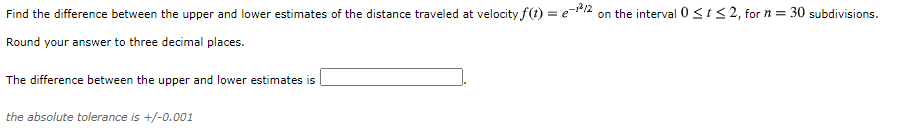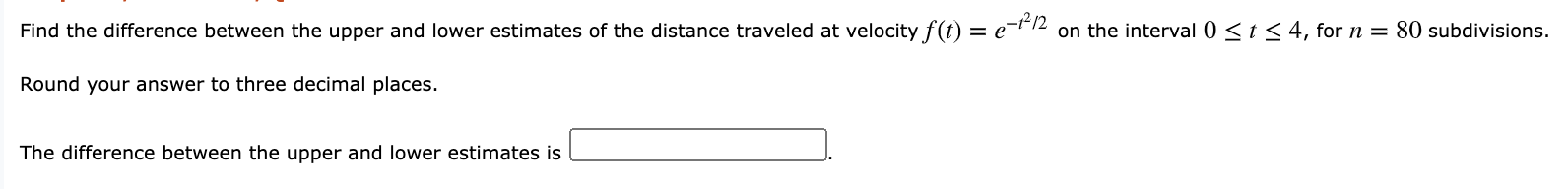# Question Solved1 Answer1.2. Find the difference between the upper and lower estimates of the distance traveled at velocity f(t) = (-1/2 on the interval 0 St<2, for n = 30 subdivisions. Round your answer to three decimal places. The difference between the upper and lower estimates is the absolute tolerance is +/-0.001 Find the difference between the upper and lower estimates of the distance traveled at velocity f(t) = e=112 on the interval 0 st 54, for n = 80 subdivisions. Round your answer to three decimal places. The difference between the upper and lower estimates is1.2.Transcribed Image Text: Find the difference between the upper and lower estimates of the distance traveled at velocity f(t) = (-1/2 on the interval 0 St<2, for n = 30 subdivisions. Round your answer to three decimal places. The difference between the upper and lower estimates is the absolute tolerance is +/-0.001 Find the difference between the upper and lower estimates of the distance traveled at velocity f(t) = e=112 on the interval 0 st 54, for n = 80 subdivisions. Round your answer to three decimal places. The difference between the upper and lower estimates is
More
Transcribed Image Text: Find the difference between the upper and lower estimates of the distance traveled at velocity f(t) = (-1/2 on the interval 0 St<2, for n = 30 subdivisions. Round your answer to three decimal places. The difference between the upper and lower estimates is the absolute tolerance is +/-0.001 Find the difference between the upper and lower estimates of the distance traveled at velocity f(t) = e=112 on the interval 0 st 54, for n = 80 subdivisions. Round your answer to three decimal places. The difference between the upper and lower estimates is